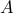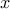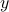# Block Splitting for Distributed Optimization

N. Parikh and S. Boyd

Mathematical Programming Computation, 6(1):77-102, 2014.
A shorter, preliminary version appeared as a NIPS workshop paper.

This paper describes a general purpose method for solving convex optimization problems in a distributed computing environment. In particular, if the problem data includes a large linear operator or matrix, the method allows for handling each subblock ofon a separate machine. The approach works as follows. First, we define a canonical problem form called graph form, in which we have two sets of variablesandrelated by a linear operator, such that the objective function is separable across these two sets of variables. Many problems are easily expressed in graph form, including cone programs and a wide variety of regularized loss minimization problems from statistics, like logistic regression, the support vector machine, and the lasso. Next, we describe graph projection splitting, a form of Douglas-Rachford splitting or the alternating direction method of multipliers, to solve graph form problems serially. Finally, we derive a distributed block splitting algorithm based on graph projection splitting. In a statistical or machine learning context, this allows for training models exactly with a huge number of both training examples and features, such that each processor handles only a subset of both. To the best of our knowledge, this is the only general purpose method with this property. We present several numerical experiments in both the serial and distributed settings.